# 生信代码：传染病传播模拟↑↑↑   关注选刊说   ↑↑↑

`` install.packages("EpiModel",dependencies = TRUE)``

1. DCMs，确定性隔间模型求解连续时间分析型流行病系统的微分方程。这些模型是确定性的，因为它们的解是输入参数和初始条件的固定数学函数，在疾病和人口过渡过程中没有随机变异性。这些模型是划分的，因为它们将人群分成代表离散疾病状态的组(例如，易感和感染)，并进一步分析影响疾病传播的人口统计学、生物学和行为特征。与下面介绍的随机模型相比，人口中的个体并不是离散表示的。其控制模型又分为三种：Susceptible-Infected (SI)，Susceptible-Infectious-Recovered(SIR)，Susceptible-Infected-Susceptible (SIS)。三个模型的调用需要用到函数control.dcm。其参数就是type和nsteps。另外呢，在启动DCM需要启动参数，行动速率和传播速率等基础参数。

1）构建一个SI模型，也就是易感和感人的人群模型，模型的公式及相关参数如下：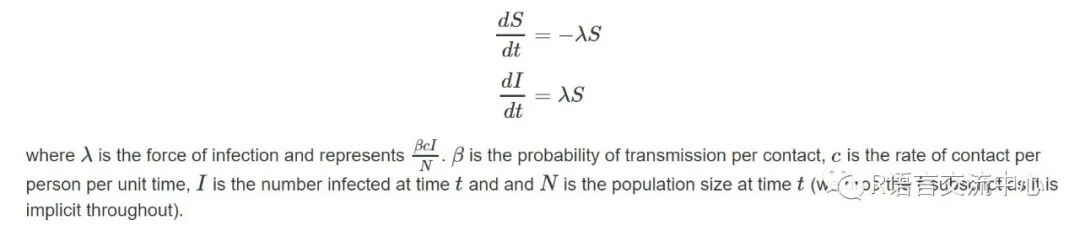``param <- param.dcm(inf.prob = 0.2,act.rate = 0.25)``init <- init.dcm(s.num = 500, i.num = 1)``control <- control.dcm(type ="SI", nsteps = 500)``mod <- dcm(param, init, control)``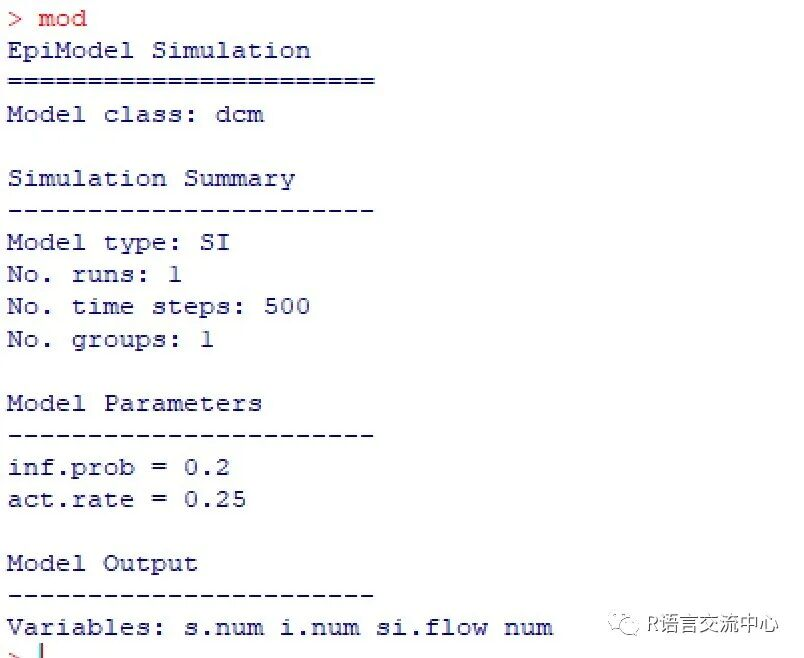s.num 每个求解时间步长的易感染人群的大小。

i.num 每个求解时间步长的感染人群的大小。

Si.flow 每个时间步长从S移动到I的人数。

``plot(mod)``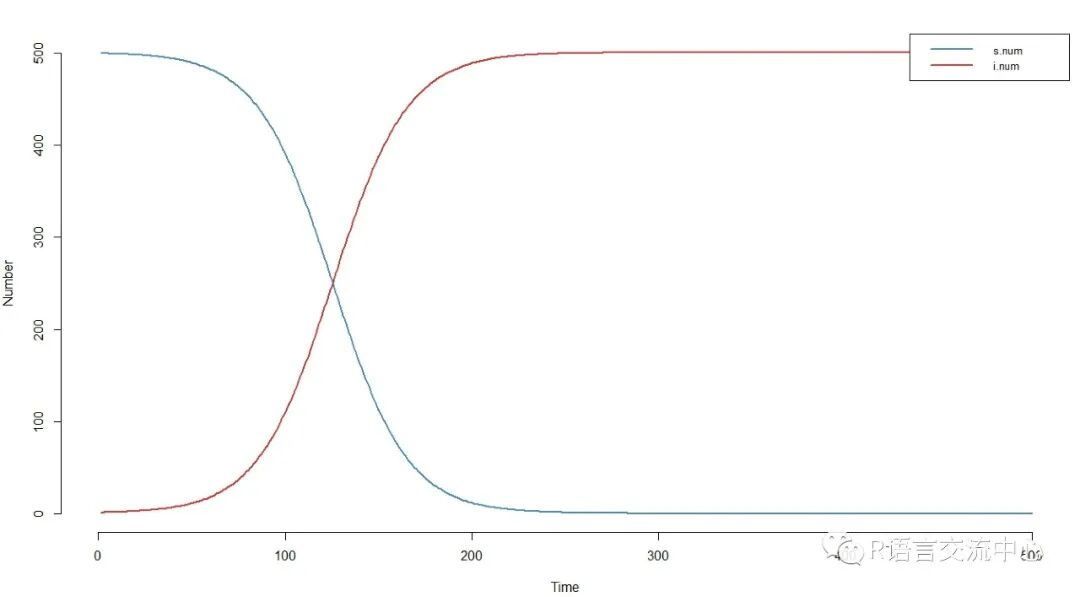2）SIR模型中多了一个终身恢复的个体：受感染的个体从疾病过渡到终身恢复状态，在这个状态中他们再也不会受感染。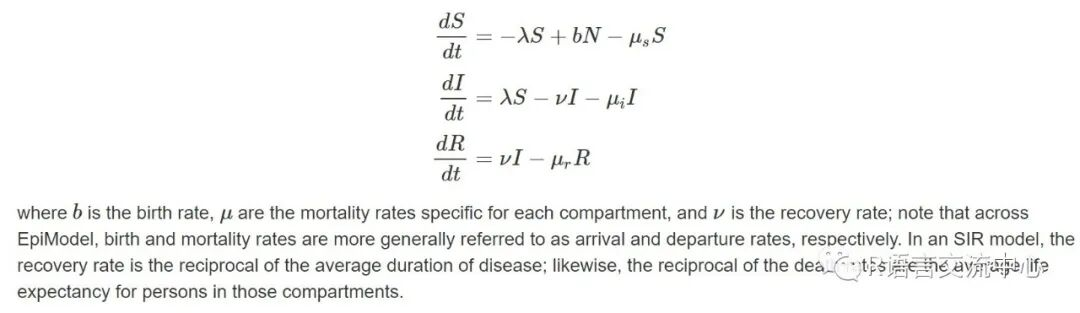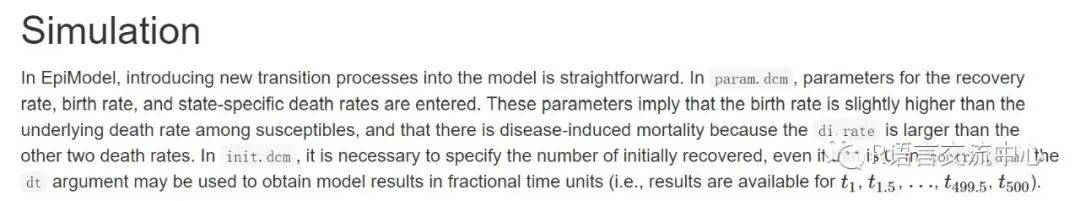``param <- param.dcm(inf.prob = 0.2,act.rate = 1, rec.rate = 1/20,``                   a.rate = 1/95, ds.rate =1/100, di.rate = 1/80, dr.rate = 1/100)``init <- init.dcm(s.num = 1000, i.num =1, r.num = 0)``control <- control.dcm(type ="SIR", nsteps = 500, dt = 0.5)``mod <- dcm(param, init, control)``

``par(mar = c(3.2, 3, 2, 1), mgp = c(2, 1,0), mfrow = c(1, 2))``plot(mod, popfrac = FALSE, alpha = 0.5,``    lwd = 4, main = "Compartment Sizes")``plot(mod, y = "si.flow", lwd = 4,col = "firebrick",``    main = "Disease Incidence", legend = "n")``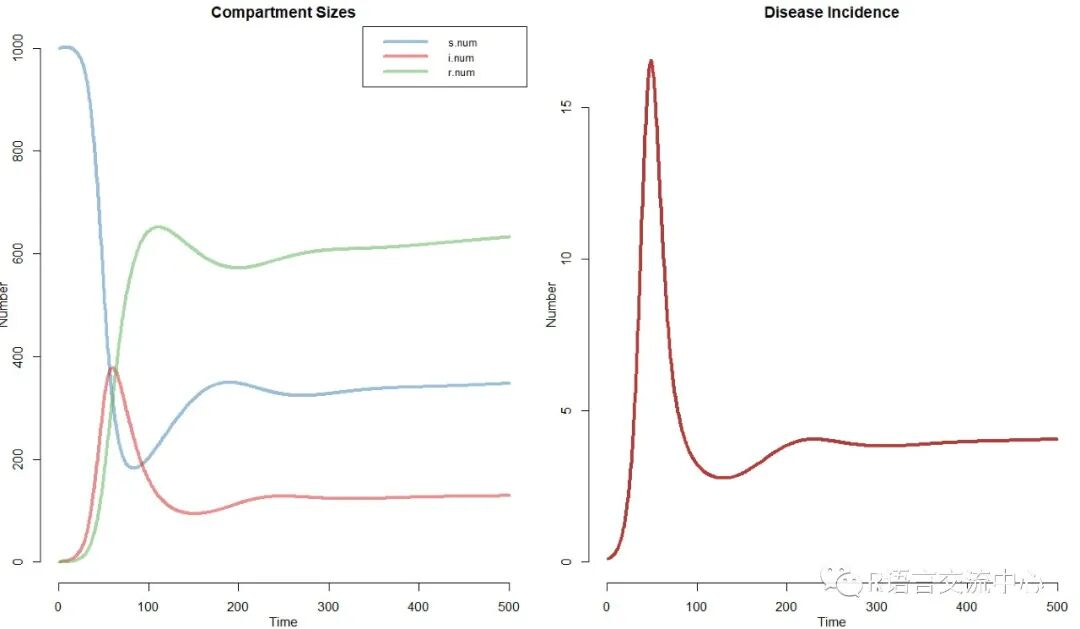``par(mfrow = c(1, 1))``comp_plot(mod, at = 50, digits = 1)``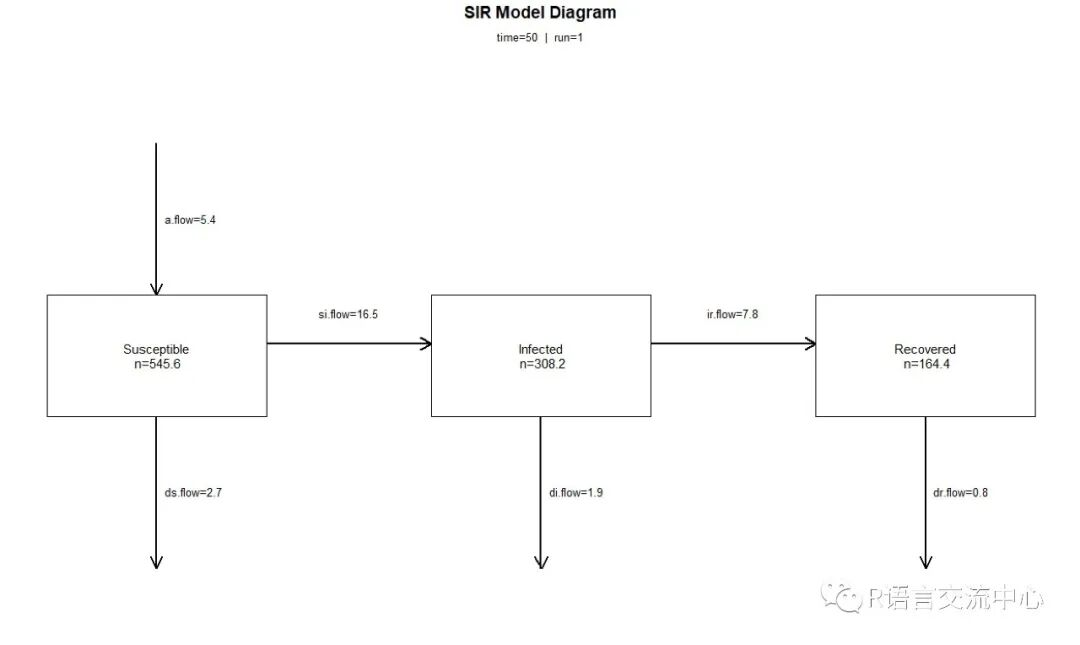3）SIS指的感染人群有转化为易感人群的模型，其中一个例子是细菌性性传播感染，如淋病。具体的模型如下：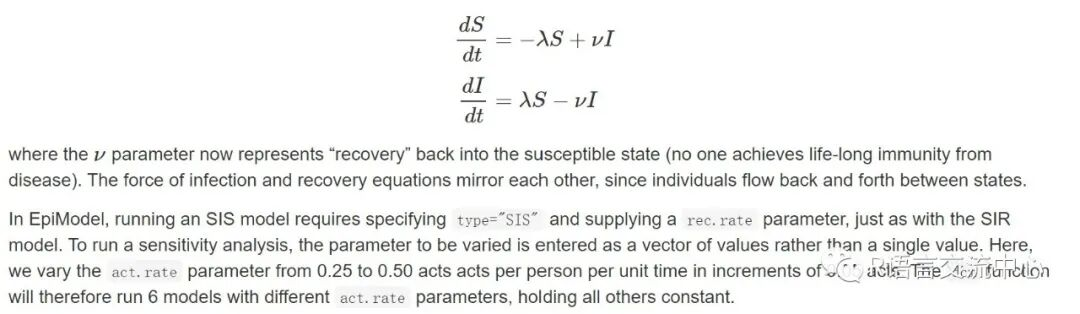``param <- param.dcm(inf.prob = 0.2,act.rate = seq(0.25, 0.5, 0.05), rec.rate = 0.02)``init <- init.dcm(s.num = 500, i.num = 1)``control <- control.dcm(type ="SIS", nsteps = 350)``mod <- dcm(param, init, control)``head(as.data.frame(mod, run = 5))``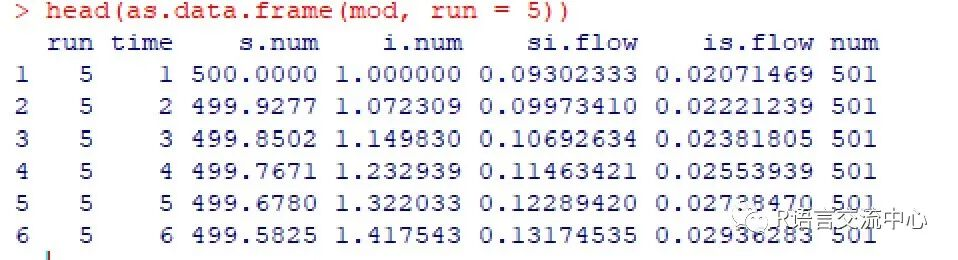``par(mfrow = c(1,2), mar = c(3.2,3,2.5,1))``plot(mod, alpha = 1, main = "Disease Prevalence")``plot(mod, y = "si.flow", col ="Greens", alpha = 0.8, main = "Disease Incidence")``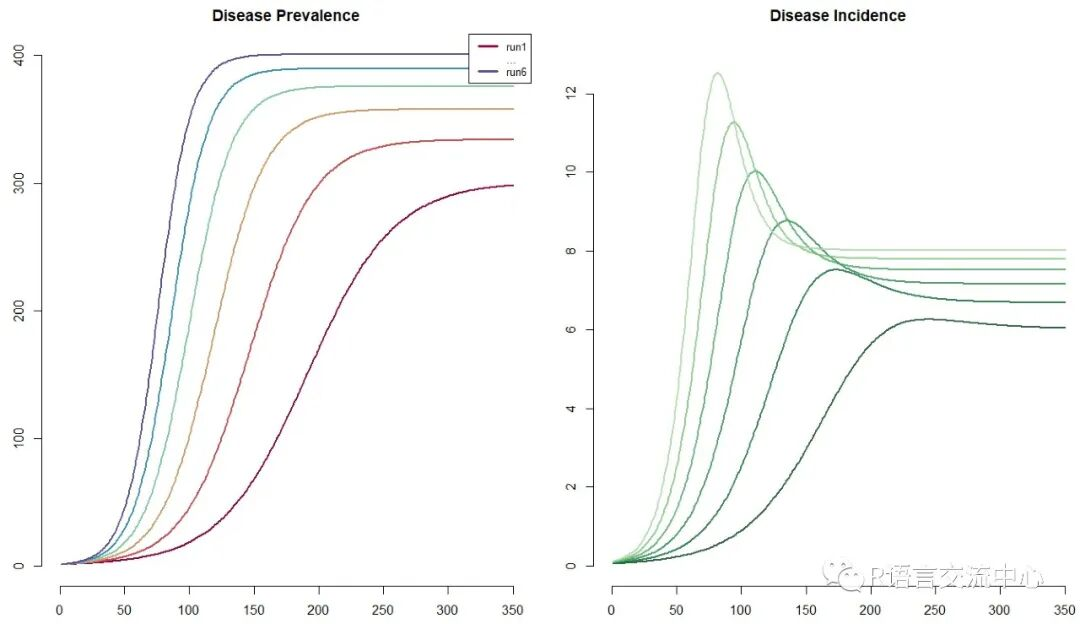``par(mfrow = c(1,2), mar = c(3.2,3,2.5,1))``plot(mod, alpha = 1, main = "DiseasePrevalence")``plot(mod, col = rainbow(3), lty = rep(1:2,each = 3), legend = "full")``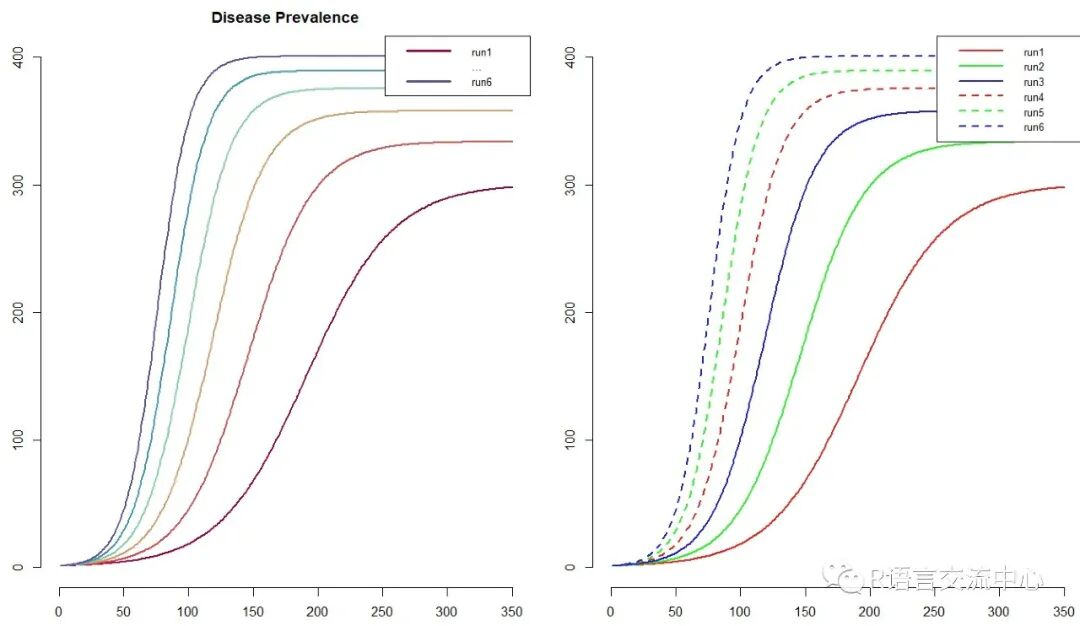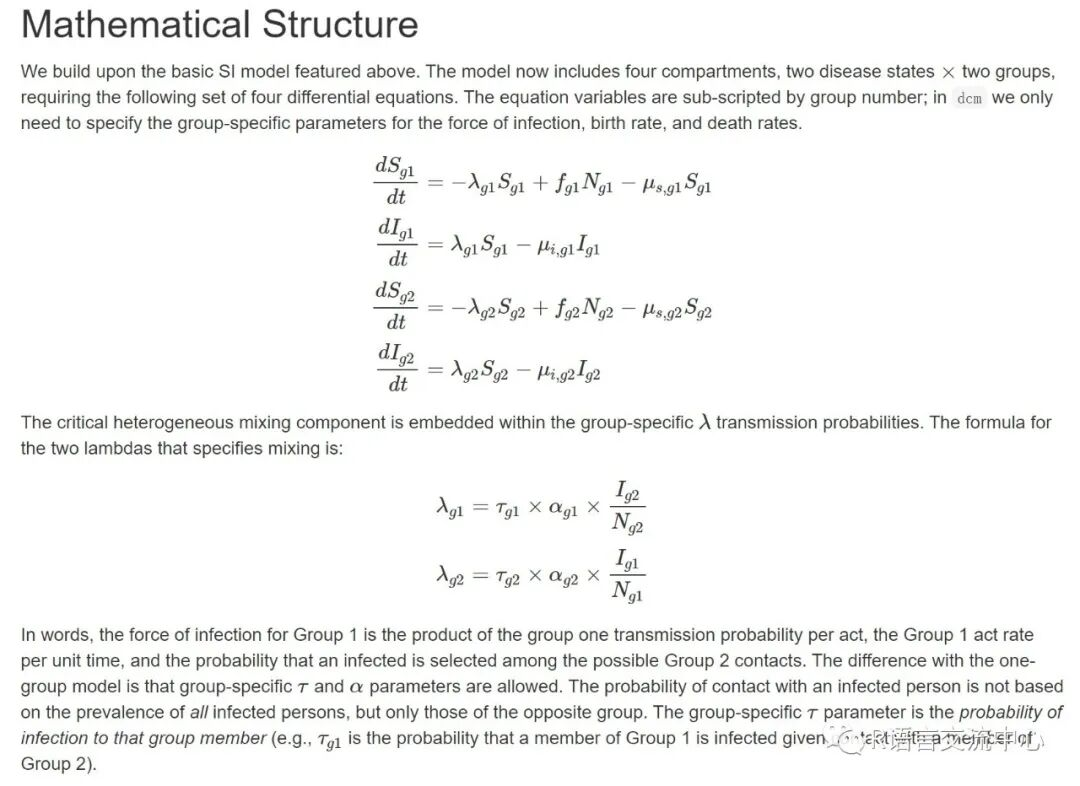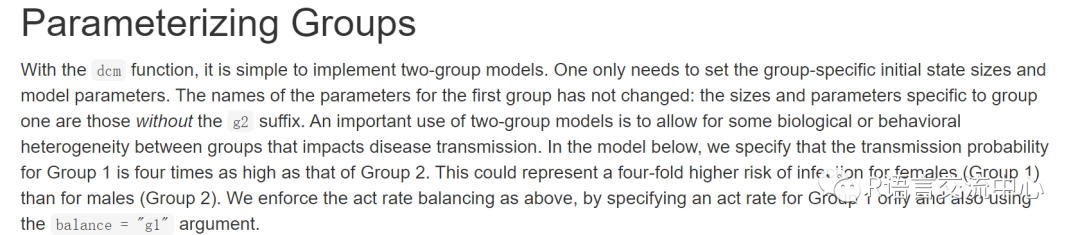``param <- param.dcm(inf.prob = 0.4,  inf.prob.g2 = 0.1, act.rate = 0.25, balance ="g1",``                   a.rate = 1/100, a.rate.g2 =NA, ds.rate = 1/100, ds.rate.g2 = 1/100,``                   di.rate = 1/50, di.rate.g2 =1/50)``init <- init.dcm(s.num = 500, i.num = 1,s.num.g2 = 500, i.num.g2 = 0)``control <- control.dcm(type ="SI", nsteps = 500)``mod <- dcm(param, init, control)``plot(mod)``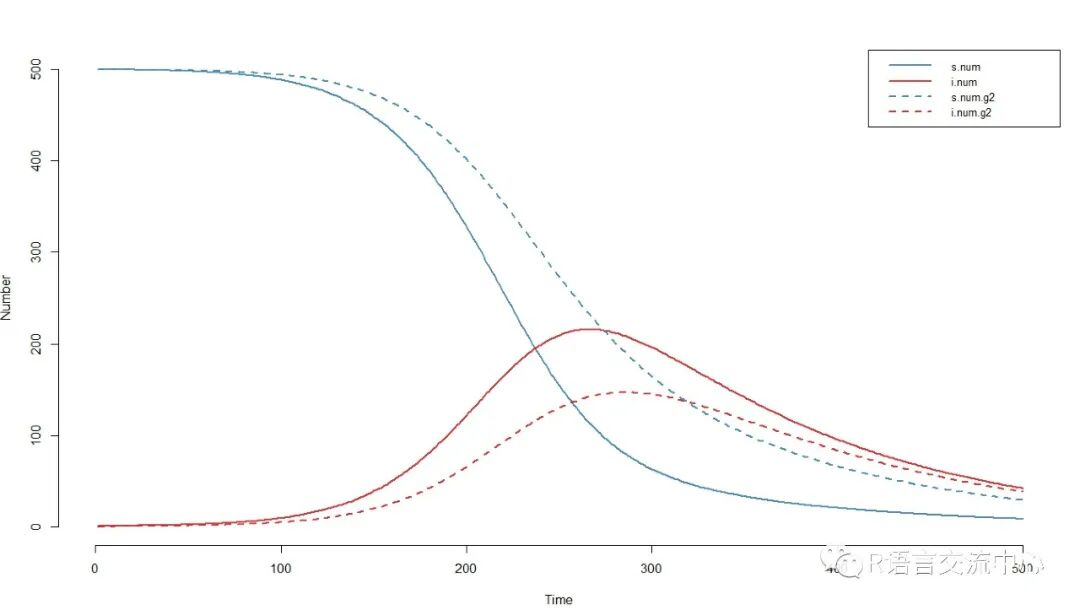2. ICMs，随机个体接触模型主要和DCMs存在以下的几个差异：参数是随机抽取的:这些是随机模型，其中控制状态间转移的所有比率和风险都是随机抽取的，它们来自由这些比率或概率总结的分布，包括正态分布、泊松分布和二项分布；时间是离散的:ICMs是在离散时间内模拟的，而DCMs是在连续时间内模拟的。在离散时间中，时间步内的所有事件都是作为一系列过程发生的，因为不存在独立于其他事件的瞬时事件发生，在连续时间模型中是可能的。如果时间步长的单位很大，这可能会在建模中引入一些人为因素，因为在时间步内可能发生的转换不一定被认为是独立的。一般来说，在模拟任何单位时间较长的离散时间模型时都需要注意；单位是个体:ICMs模拟疾病在模拟的个体可识别元素种群上的传播;相反，DCMs将总体视为一个可以无限分割的聚合整体，其中的单个元素既不能被识别，也不能用于建模。如果随机模拟发生在一个足够大的种群上，那么这种影响相对较小，但是对于基于个人的模拟，有一些关键的建模注意事项，将在下面的示例中进行回顾。

ICMs和DCMs的语法基本一致，只是在输出的结果中，存在差异，ICMs标准的绘图输出包括跨模拟的平均值以及值的四分位范围;默认情况下两者都是平滑值。我们直接看下SI实例：

``param <- param.icm(inf.prob = 0.2,act.rate = 0.25)``init <- init.icm(s.num = 500, i.num = 1)``control <- control.icm(type ="SI", nsims = 10, nsteps = 300)``mod <- icm(param, init, control)``plot(mod)``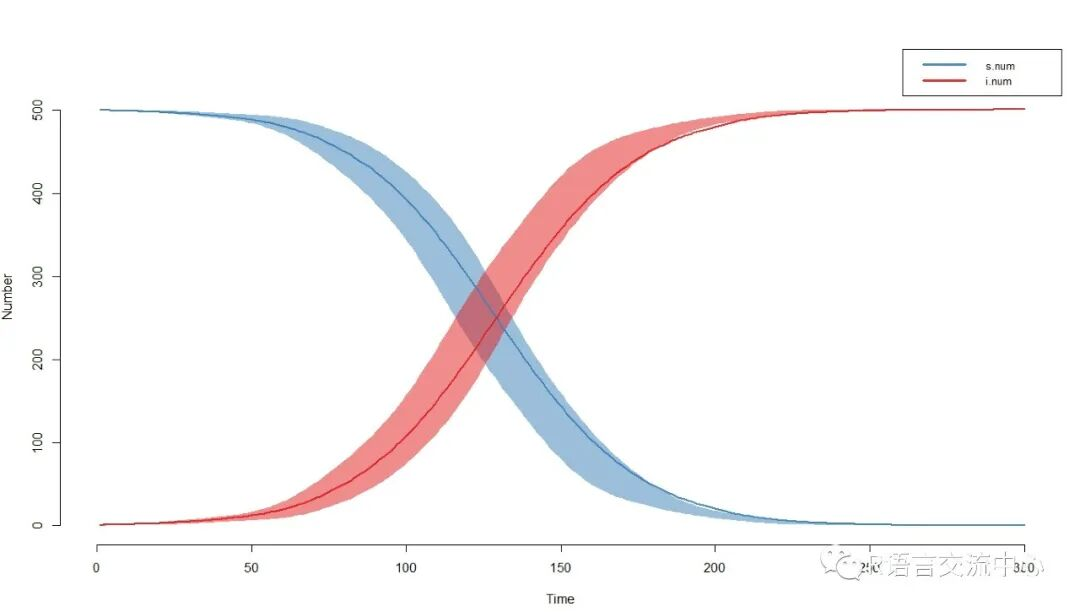``plot(mod, y = "i.num", sim.lines= TRUE, mean.smooth = FALSE, qnts.smooth = FALSE)``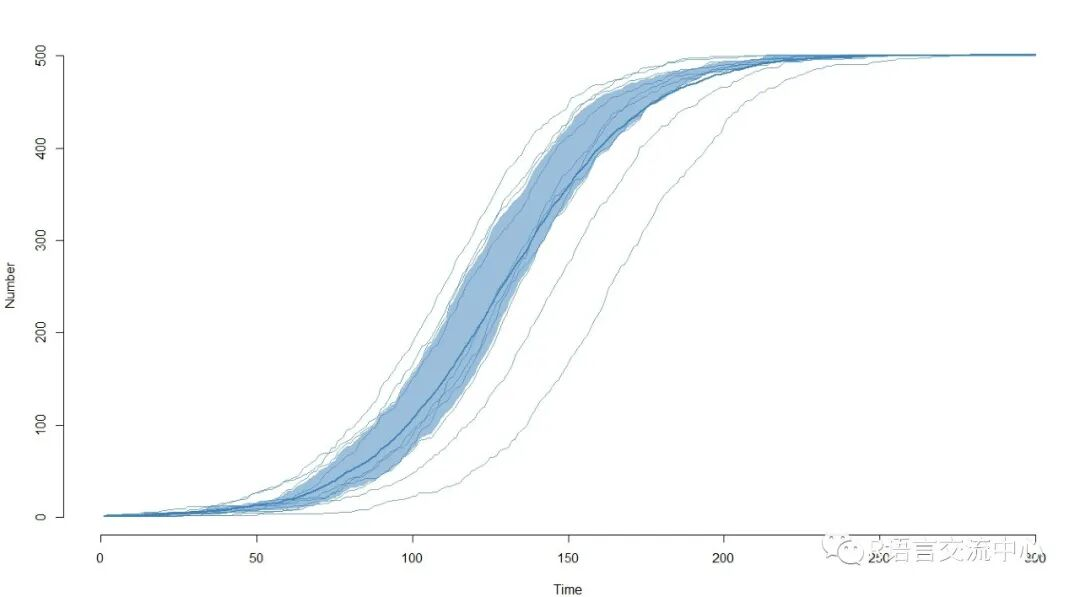3. 随机网络模型是两个节点之间连边与否不再是确定的事情，而是根据一个概率决定。从某种意义上讲，规则网络和随机网络是两个极端，而复杂网络处于两者之间。此部分仍然需要对应的模型参数，首先它也具有了DCMs和ICMs的控制模型SI，SIR和SIS。网络模型的一个关键区别在于，网络动力学和疾病传播动力学这两个动态过程是被视为独立的或者相互依赖的。独立的网络模型假设疾病模拟对时间网络的结构没有影响，尽管时间网络的结构肯定会影响疾病。依赖网络模型允许流行病学和人口统计过程影响网络结构。例如血清监测——疾病状况影响伴侣选择——和人口统计过程(出生、死亡和迁移)——接触网络过程必须适应人口规模和组成的变化。其中主要包括三个主要的功能函数：netest估计了动态伙伴关系网络的生成模型；netdx模拟与netest模型拟合一致的动态网络的时间序列，以检查模型拟合是否符合以自我为中心的目标统计；netsim采用netest网络模型拟合的随机流行病模型。对于独立模型，在每次疫情模拟开始时对全动态网络进行模拟。对于相关模型，在每个时间步长的情况下，网络被重新模拟成变化的种群大小和变化的节点属性的函数。说了这么多，接下来我们直接从实例出发：

#构建一个独立无向的单模网络模型

``nw <-network.initialize(n = 1000, directed = FALSE)``nw <-set.vertex.attribute(nw, "race", rep(0:1, each = 500))``formation <-~edges + nodefactor("race") + nodematch("race") +concurrent``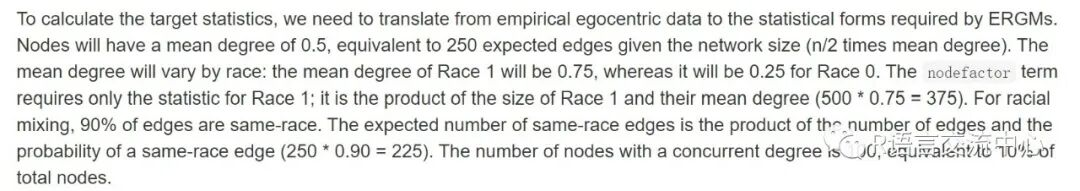``target.stats <- c(250, 375, 225, 100)``

``coef.diss <-dissolution_coefs(dissolution = ~offset(edges), duration = 25)``coef.diss``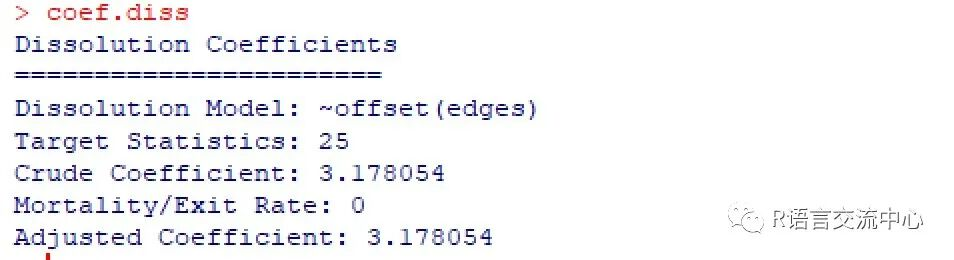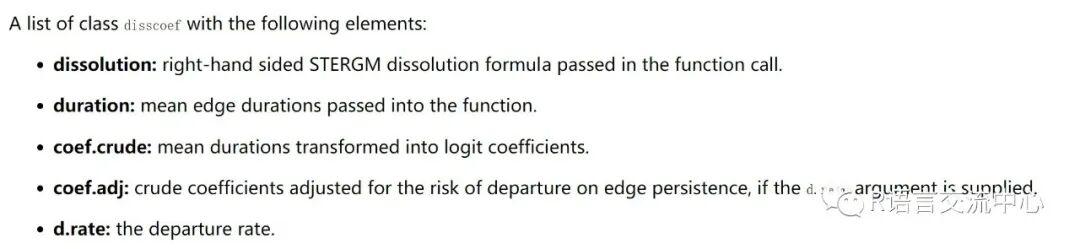``#模型评估``est1 <- netest(nw, formation,target.stats, coef.diss, edapprox = TRUE)``#模型诊断``dx <- netdx(est1, nsims = 5, nsteps =500,``           nwstats.formula = ~edges + nodefactor("race", base = 0) +``                              nodematch("race") + concurrent)``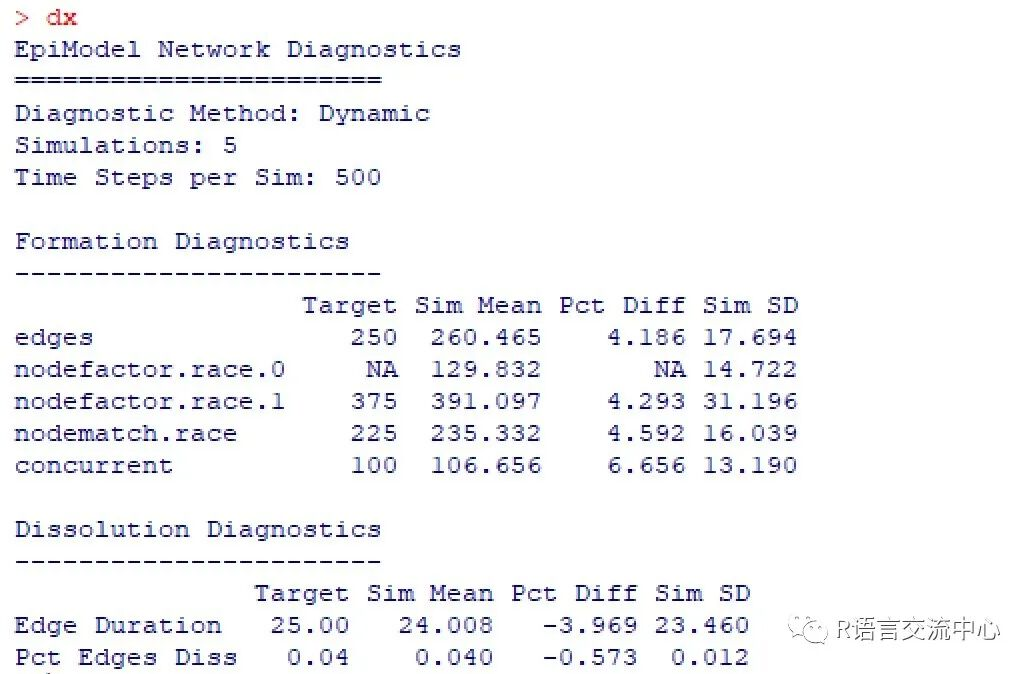``par(mar = c(3,3,1,1), mgp = c(2,1,0))``plot(dx)``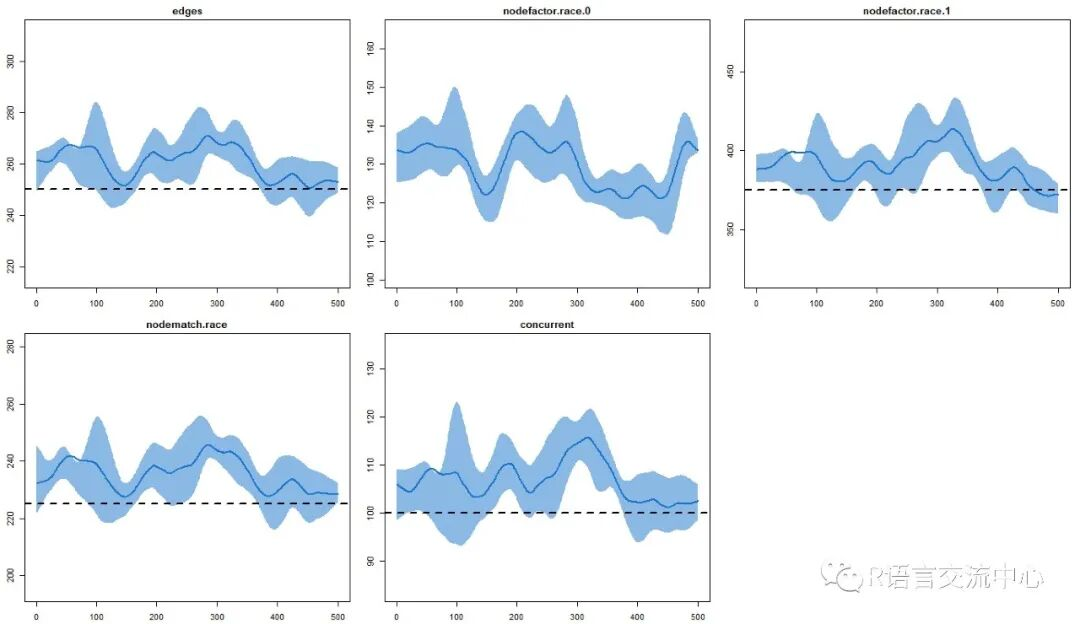#绘制分解模型

``par(mfrow = c(1, 2))``plot(dx, type = "duration")``plot(dx, type = "dissolution")``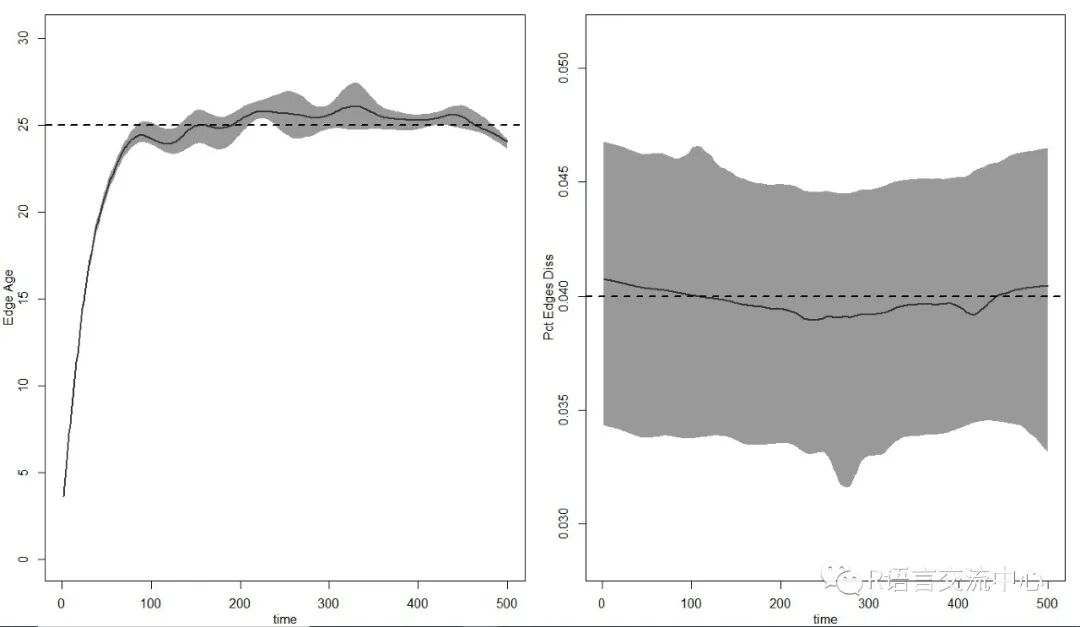``#模型参数设置``param <- param.net(inf.prob = 0.1,act.rate = 5, rec.rate = 0.02)``status.vector <- c(rbinom(500, 1, 0.1),rep(0, 500))``status.vector <- ifelse(status.vector ==1, "i", "s")``init <- init.net(status.vector =status.vector)``control <- control.net(type ="SIS", nsteps = 500, nsims = 10, epi.by = "race")``#模型构建``sim1 <- netsim(est1, param, init,control)``#数据结果``head(as.data.frame(sim1), 10)``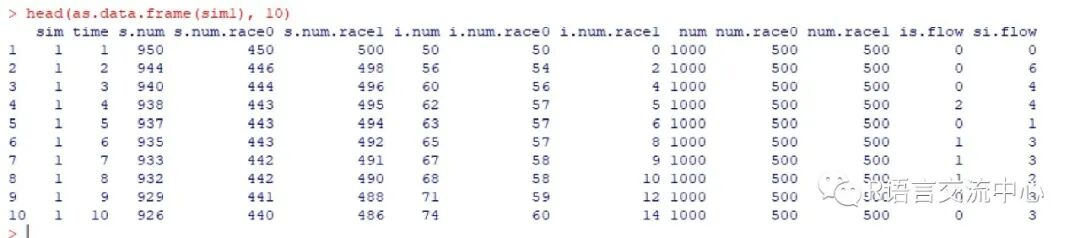``#获得网络状态``nw <- get_network(sim1, sim = 1)``#获得每个时间点的时间状态``get_transmat(sim1, sim = 1)``#绘制任意时间点的网络状态``par(mfrow = c(1,2), mar = c(0,0,1,0))``plot(sim1, type = "network", at =1, col.status = TRUE,``    main = "Prevalence at t1")``plot(sim1, type = "network", at =500, col.status = TRUE,``    main = "Prevalence at t500")``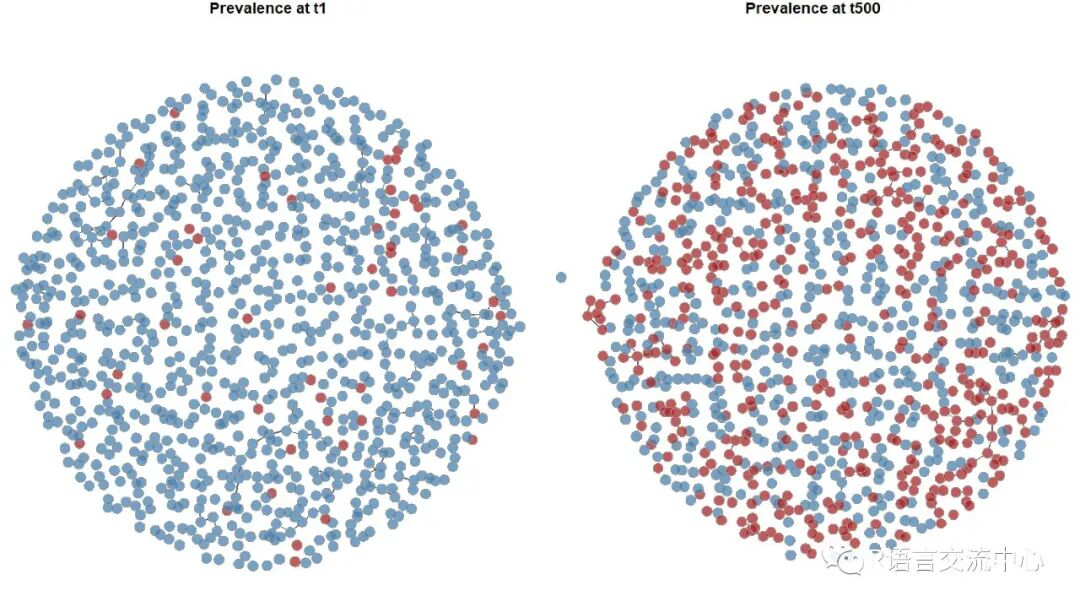``#模型参数``param <- param.net(inf.prob = 0.3,inf.prob.m2 = 0.1,``                   a.rate = 0.005, a.rate.m2 =NA,``                   ds.rate = 0.005, ds.rate.m2= 0.005,``                   di.rate = 0.005, di.rate.m2= 0.005)``init <- init.net(i.num = 50, i.num.m2 =50)``control <- control.net(type ="SI", nsims = 5, nsteps = 500,``                       nwstats.formula = ~edges+ meandeg, delete.nodes = TRUE)``num.m1 <- 500``num.m2 <- 500``nw <- network.initialize(num.m1 +num.m2, bipartite = num.m1, directed = FALSE)``formation <- ~edges + b1degree(0:1) +b2degree(0:1)``target.stats <- c(330, 200, 275, 240,205)``coef.diss <-dissolution_coefs(dissolution = ~offset(edges), duration = 25,``                               d.rate = 0.005)``#模型构建``est2 <- netest(nw, formation,target.stats, coef.diss)``sim2 <- netsim(est2, param, init,control)``#可视化结果``plot(sim2, popfrac = FALSE)``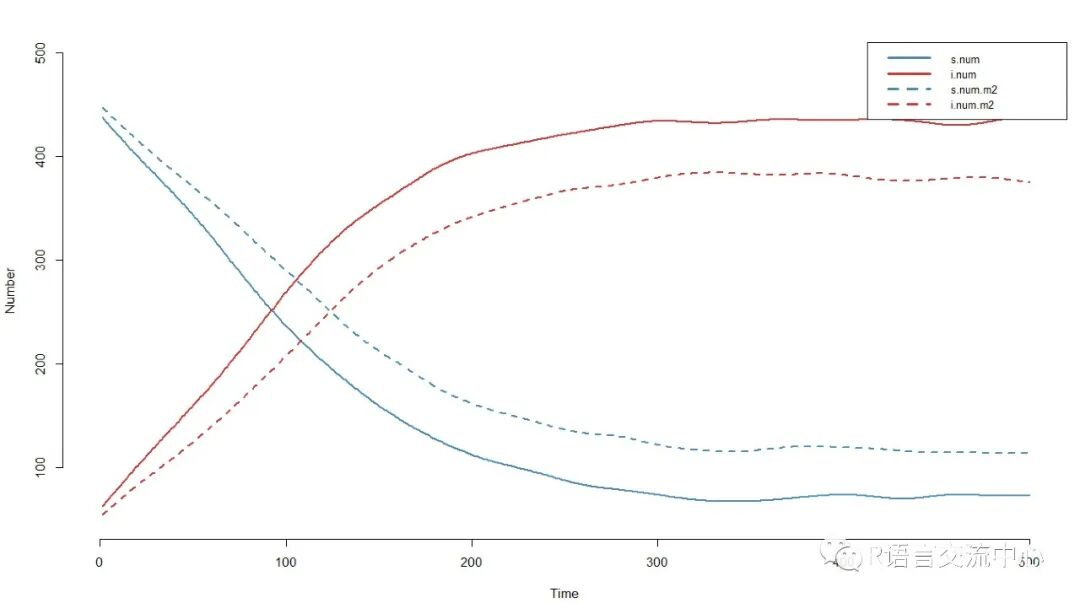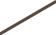## 这本连续7分多的SCI，3个月接受，年发文2000篇左右

2021-1-17 18:56:02

## 超实用R包，一行代码输出两个数据集间的各项差异

2021-1-17 19:24:48